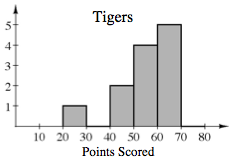### Home > CC2MN > Chapter 10 > Lesson 10.2.1 > Problem10-23

10-23.

The Tigers and the Panthers are in the same league and each play $12$ games during a basketball season. The points they scored each game are shown in the table below.

 Tigers Panthers $46$ $62$ $51$ $57$ $45$ $54$ $61$ $25$ $50$ $66$ $63$ $68$ $54$ $61$ $74$ $78$ $52$ $57$ $69$ $48$ $28$ $51$ $53$ $23$
1. Make a histogram for each team. Each bin should have an interval of $10$ points ($20-30, 30-40$, etc.).

For each team, start by dividing their points by bins (meaning, count how many games had between 20 and 30 points, 30 and 40 points, etc.). Then use this information to make the histograms.

Below is the histogram for the Tigers. Use it to help you make a histogram for the Panthers.2. Calculate the mean and mean absolute deviation for each team.

The mean is the average of the points scored each game.
To find the mean for the Tigers, sum the points of all the games and divide by the number of games.
$\frac{46+62+51+57+45+54+61+25+50+66+63+68}{12}=\ 54\ \text{points per game}$
Use this as a guide to find the mean for the Panthers.

To find the mean absolute deviation, you must first find the absolute difference between each data point and the mean.
Then find the mean of these differences.

3. Why was finding the mean for the Tigers not the best measure of center for the data?

Look again at the shape of the distribution. Was it roughly symmetrical or is the histogram skewed?

4. Write a complete explanation for which team you think is “better” and why. Be sure you compare the center, shape, spread, and outliers.

It will be necessary to consider factors other than the mean points scored.
If a team is inconsistent, then the other team is likely ''better.'' How can you measure consistency?

Both teams have the same average, but the Tigers have a lower mean absolute deviation. They are more consistent.
The Panthers have some very good scores, but they also have some very bad scores.